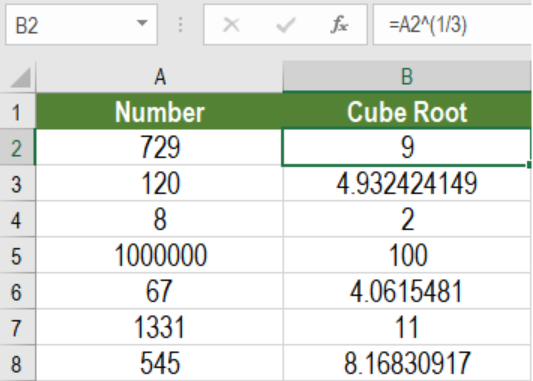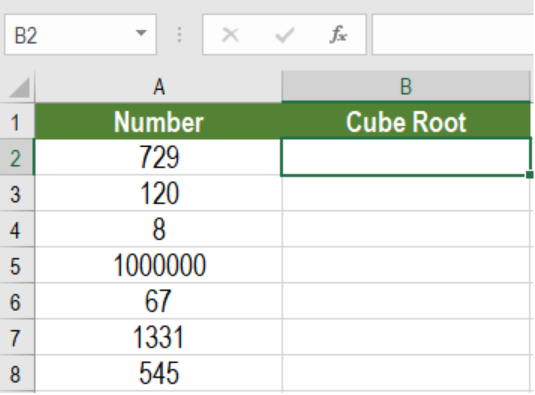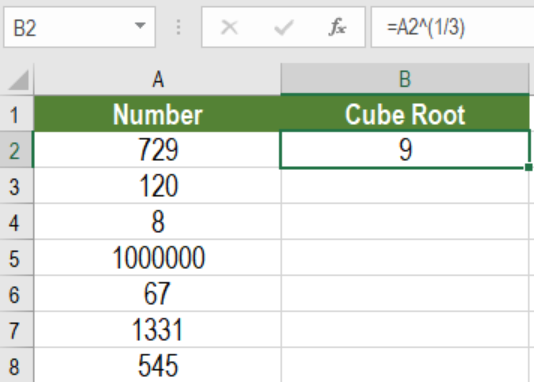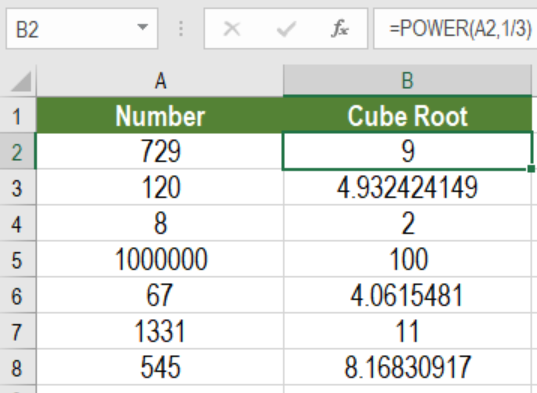Get instant live expert help with Excel or Google Sheets“My Excelchat expert helped me in less than 20 minutes, saving me what would have been 5 hours of work!”

#### Post your problem and you’ll get Expert help in seconds.

Your message must be at least 40 characters
Our professional Expert are available now. Your privacy is guaranteed.

# How to Calculate the Cube Root of a Number in Excel

Excel offers some great tools for mathematical calculations. One of these is to calculate the cube root of a number. To find the cube root of a number, we can use the caret operator (^) or the POWER function. In this tutorial, we will learn how to calculate the cube root of a number in Excel.Figure 1. Example of How to Calculate the Cube Root of a Number

## Syntax to Calculate Cube Root using the Caret Operator

`=Num^(1/3)`

We can get the cube root of a number using the caret operator with the exponent 1/3. The caret operator raises the number to its 1/3 power which is equivalent to its cube root.

## Setting Up Data

The following example contains some sample numbers. Column A has these numbers.Figure 2. The Sample Data Set

To get the cube roots in column B using the caret operator:

• We need to go to cell B2.
• Assign the formula `=A2^(1/3) `to cell B2.
• Press Enter.Figure 3. Example of Applying the Caret Operator to the Formula

• Drag the formula from cells B2 to B8 to copy the formula to the entire column.

This will show the cube roots of the number in column B.

## Syntax to Calculate Cube Root using the POWER Function

`=POWER(Num, 1/3)`

We can achieve the same results as before using the POWER function. The POWER function takes two arguments. The first one is the number which we want to find the cube root for. The second argument here will be 1/3, which will return the cube root of the number.

To calculate the cube roots of the numbers in the previous example using the POWER function:

• We need to go to cell B2.
• Assign the formula `=POWER(A2,1/3) `to cell B2.
• Press Enter.
• Drag the formula from cells B2 to B8 to copy the formula to the entire column.Figure 4. Applying the POWER function to Find the Cube Root of a Number

This will also show the cube roots of the number in column B.

Most of the time, the problem you will need to solve will be more complex than a simple application of a formula or function. If you want to save hours of research and frustration, try our live Excelchat service! Our Excel Experts are available 24/7 to answer any Excel question you may have. We guarantee a connection within 30 seconds and a customized solution within 20 minutes.

Solution examplesI need help with solving equations using separate columns. For example. One column has pH values going from 0-14 and the column next to it has the equation [H+] = 10^-pH with it's corresponding values.
Solved by I. J. in 18 mins